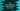# C++ program to find the smallest and the largest elements in an array## Smallest and largest elements in a C++ array :

In this program, we will find the smallest and largest elements in an array using C++. We will take the array elements as inputs from the user and find out the smallest and largest.

### Algorithm :

The following algorithm we will use to solve this problem :

1. Ask the user to enter the total number of elements.
2. Create one array with size as the total number of elements that user has entered. This array is to hold the user input elements.
3. Create two variables to hold the largest and smallest values of the array. Assign both the first array element’s value.
4. Iterate through the array elements one by one.
5. Check for each element if it is smaller than the current smallest value or larger than the current largest value.
6. If the above step is true, update the smallest and the largest value variable.

### C++ program :

``````#include <iostream>
#include <iomanip>
using namespace std;

int main()
{
int count;

cout << "Enter total number of elements :" << endl;
cin >> count;

int values[count];

for (int i = 0; i < count; i++)
{
cout << "Enter the number for position " << i + 1 << endl;
cin >> values[i];
}

int smallest = values;
int largest = values;

for (int i = 1; i < count; i++) {

if (values[i] > largest)
{
largest = values[i];
}

if (values[i] < smallest)
{
smallest = values[i];
}
}

cout << "Smallest number is : " << smallest << endl;
cout << "Largest number is : " << largest << endl;
}``````

### Explanation :

1. Ask the user to enter the total number of numbers. Store it on variable count.
2. values is an array of size count. This array will hold all user input numbers.
3. The first for loop takes the user input numbers one by one and enters them in values array.
4. Assign the first number of values as the smallest and largest of all.
5. The second loop runs from the second element of the array since we have assigned the first element as the current largest and smallest element.
6. Check each element of the array if it is greater than the current largest or smaller than the current smallest element.
7. Update the smallest and the largest element if the above step is true.
8. Finally, print both smallest and largest numbers.

### Sample output :

``````Enter total number of elements :
4
Enter the number for position 1
1
Enter the number for position 2
2
Enter the number for position 3
3
Enter the number for position 4
4
Smallest number is : 1
Largest number is : 4

Enter total number of elements :
5
Enter the number for position 1
12
Enter the number for position 2
334
Enter the number for position 3
102
Enter the number for position 4
2220
Enter the number for position 5
1
Smallest number is : 1
Largest number is : 2220``````